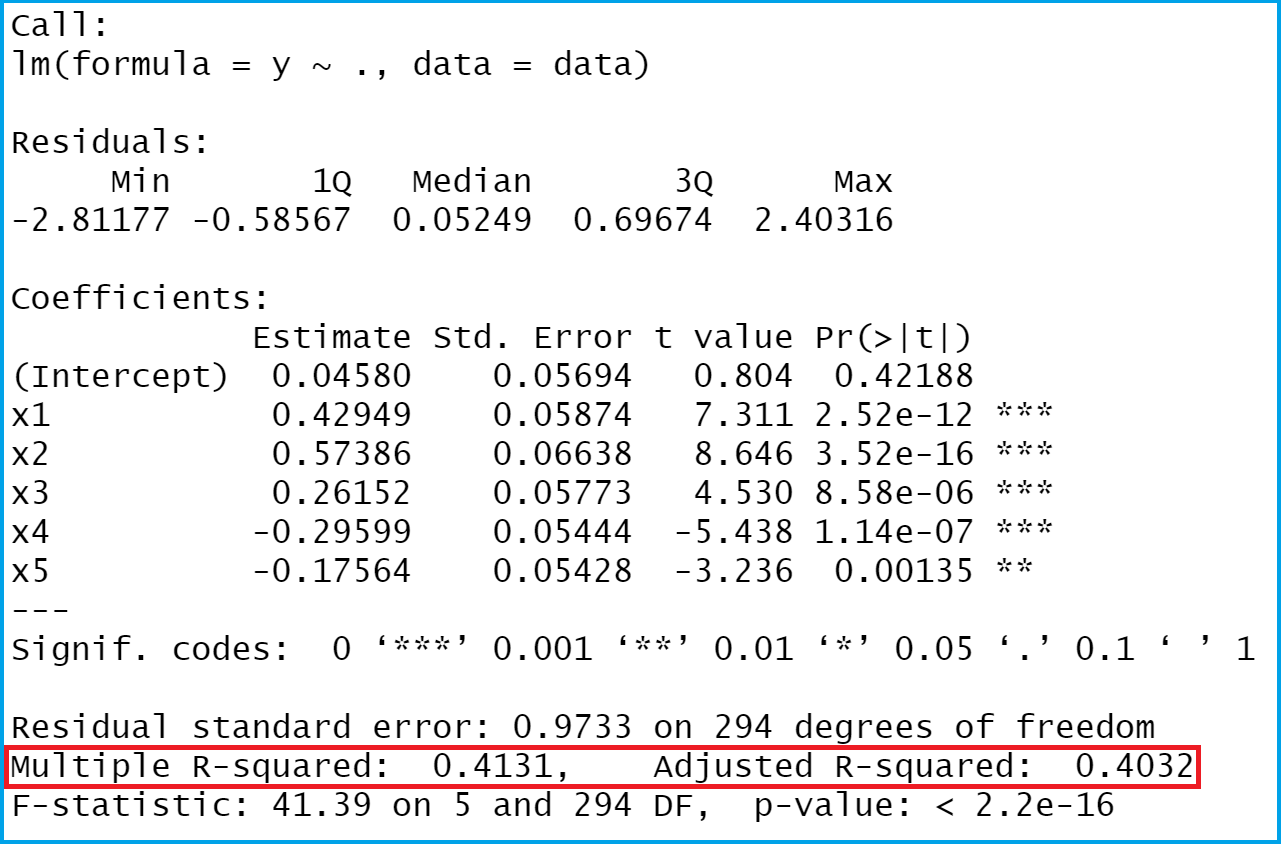# Extract Multiple & Adjusted R-Squared from Linear Regression Model in R (2 Examples)

In this tutorial you’ll learn how to return multiple and adjusted R-squared in the R programming language.

The tutorial is structured as follows:

Let’s get started!

## Example Data

First, we have to create some example data:

```set.seed(96149)                            # Create randomly distributed data
x1 <- rnorm(300)
x2 <- rnorm(300) - 0.1 * x1
x3 <- rnorm(300) + 0.1 * x1 - 0.5 * x2
x4 <- rnorm(300) - 0.4 * x2 - 0.1 * x3
x5 <- rnorm(300) + 0.1 * x1 - 0.2 * x3
y <- rnorm(300) + 0.5 * x1 + 0.5 * x2 + 0.15 * x3 - 0.4 * x4 - 0.25 * x5
data <- data.frame(y, x1, x2, x3, x4, x5)
head(data)                                 # Show first six lines
#             y          x1         x2          x3         x4         x5
# 1 -0.2553708  0.4399836  0.2144276 -0.24921404  0.7626867 -0.000145643
# 2  0.9582395  0.1866435 -0.8674311  0.56741079  0.2266811 -0.482339176
# 3  0.5354913  0.5123466  0.8521783 -0.15192973 -0.5772924  1.598023729
# 4 -0.1751357 -0.2642710 -0.6622039  0.91587607 -0.8784139  0.175314482
# 5  0.4741015 -1.2264237  1.1414974 -0.02544234 -1.4704185  2.154123548
# 6 -2.4687764 -0.4832017 -0.6963652 -0.27676098  3.5740767  1.535050595```

The previous output of the RStudio console illustrates the structure of our example data – It consists of six variables, whereby the column y is containing our outcome and the remaining columns are used as predictors.

Let’s use our data to fit a linear regression model in R:

```mod_summary <- summary(lm(y ~ ., data))    # Run linear regression model
mod_summary                                # Summary of linear regression model```The previous image shows the output of our linear regression analysis. I have marked the values we are interested in in this example in red.

## Example 1: Extracting Multiple R-squared from Linear Regression Model

This Example shows how to pull out the multiple R-squared from our output.

```mod_summary\$r.squared                      # Returning multiple R-squared
# 0.4131335```

The RStudio console shows our result: The multiple R-squared of our model is 0.4131335.

## Example 2: Extracting Adjusted R-squared from Linear Regression Model

Alternatively to the multiple R-squared, we can also extract the adjusted R-squared:

```mod_summary\$adj.r.squared                  # Returning adjusted R-squared
# 0.4031528```

The adjusted R-squared of our linear regression model is 0.4031528.

## Video, Further Resources & Summary

Do you need further info on the R programming codes of this tutorial? Then you may want to watch the following video of my YouTube channel. In the video, I’m explaining the R programming code of this tutorial.

In addition to the video, you might read the other tutorials of this homepage. I have published numerous tutorials about linear regression models already.

Subscribe to the Statistics Globe Newsletter

•Aroosa Javaid
March 10, 2021 4:36 am

hello i want to extract feature by using r square with R studio kindly guide the codding and procedure as well..thanks

•March 10, 2021 6:44 am

Hi Aroosa,

I’m not sure if I get your question. Could you please tell some more details?

Thanks!

Joachim

•Jason
April 5, 2022 6:16 pm

Hi, Joachim,

Do you have any idea how to derive outcome from summary(stan_glm). Thanks

•April 6, 2022 6:09 am

Hey Jason,

Could you explain what exactly you mean with “outcome”?

Regards,
Joachim

•Lais
June 8, 2022 9:41 am

And how can I pull if I have a list with 24 results of lm inside?

•June 8, 2022 11:09 am

Hey Lais,

Regards,
Joachim

•Fernando Figueroa
June 18, 2022 10:58 pm

Hi Joachim,

Can i get a coeficient of determination for the test predictions?

•June 20, 2022 7:38 am

Hey Fernando,

The coefficient of determination (i.e. R-Squared) is extracted in Example 1 of this tutorial. Or am I misinterpreting your question?

Regards,
Joachim

•Christian Koko
September 23, 2022 5:03 am

Hey Joachim,
I would like to know how can I get an adjusted regression. Is it the adjusted R-square?
Thanks,
Christian

•September 29, 2022 4:08 pm

Hi Christian,

Regards,
Joachim

•introduction to econometrics
June 12, 2023 9:44 am

Model Summary
Model R R Square Adjusted R Square Std. Error of the Estimate
1 .567a .321 .309 .11806
a. Predictors: (Constant), X4, X2, X1, X3

ANOVAa
Model Sum of Squares df Mean Square F Sig.
1 Regression 1.504 4 .376 26.975 .000b
Residual 3.178 228 .014
Total 4.682 232
a. Dependent Variable: WPI
b. Predictors: (Constant), X4, X2, X1, X3

Coefficientsa
Model Unstandardized Coefficients Standardized Coefficients t Sig.
B Std. Error Beta
1 (Constant) 3.200 .008 413.317 .000
X1 .040 .008 .284 5.101 .000
X2 .067 .008 .473 8.599 .000
X3 .010 .008 .069 1.231 .220
X4 .007 .008 .050 .902 .368
a. Dependent Variable: WPI

•June 12, 2023 9:59 am

Hello,

Best,
Cansu

•introduction to econometrics
June 13, 2023 12:34 pm

hello,
interpretation of the findings

•June 13, 2023 12:41 pm

Hello,

This source of Scribbr could be useful for you to interpret the results in case you are using ANOVA model.

Best,
Cansu

••June 13, 2023 12:53 pm